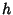## Temperature

The temperature'' of a curveis defined aswhereis the length ofandis the length of the Perimeter of the Convex Hull. The temperature of a curve is 0 only if the curve is a straight line, and increases as the curve becomes more wiggly.''